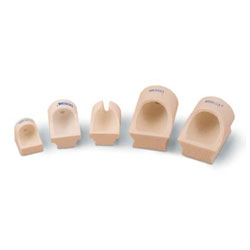### To figure out how much gold you should add to karat up:

Take your starting weight and figure exactly how much alloy is contained. Divide this weight by the alloy factor of the karat you want to go up to. This will give you the total weight of the desired karat that you will end up with. Subtract from this total weight your starting weight, and this will you you much fine gold to add.

 Example, 10K to 14K: Starting Weight 10K 350 T/oz x .5833 Total oz. alloy contained 204.155 Divide by .41667 Total amount of 14K made with this amount of alloy 489.97 T/oz Subtract starting weight -350.00 Amount of fine gold to be added 139.97 T/oz

### To figure out how much gold you should add to karat down:

Take your starting weight and figure exactly how much fine gold is contained. Divide this number by the gold factor of the karat you want to go down to. This will give you the total weight of the desired karat that you will end up with. Subtract your starting weight from this number, and that will tell you how much alloy to add.

 Example, 14K to 10K: Starting Weight 14K 500.00 T/oz x .5833 Total fine gold contained 291.65 T/oz Divide by .41667 Total amount of 10K made with this amount of fine gold 699.95 T/oz Subtract starting weight -500.00 Amount of alloy to be added 199.95 T/oz

## Simple Conversion Factor to Karat Up or Down

### Reducing Factors

To reduce karat: Find reducing factor at intersection of “Karat on Hand” and “Karat Wanted. Multiply the weight of your karat gold on hand by the reducing factor. This gives you the weight of alloy you must add to reduce to karat wanted.

 Karat Wanted
 Karat On Hand 24K 18K 14K 18K .333333 14K .714287 .285715 10K 1.400 .8000 .4000

### Reducing Factors

To raise karat: Find raising factor at intersection of “Karat on Hand” and Karat Wanted”. Multiply the weight of your karat gold on hand by the raising factor. This gives you the weight of fine gold you must add to raise to karat wanted.

 Karat Wanted
 Karat On Hand 10K 14K 14K .400 18K 1.333336 .666667